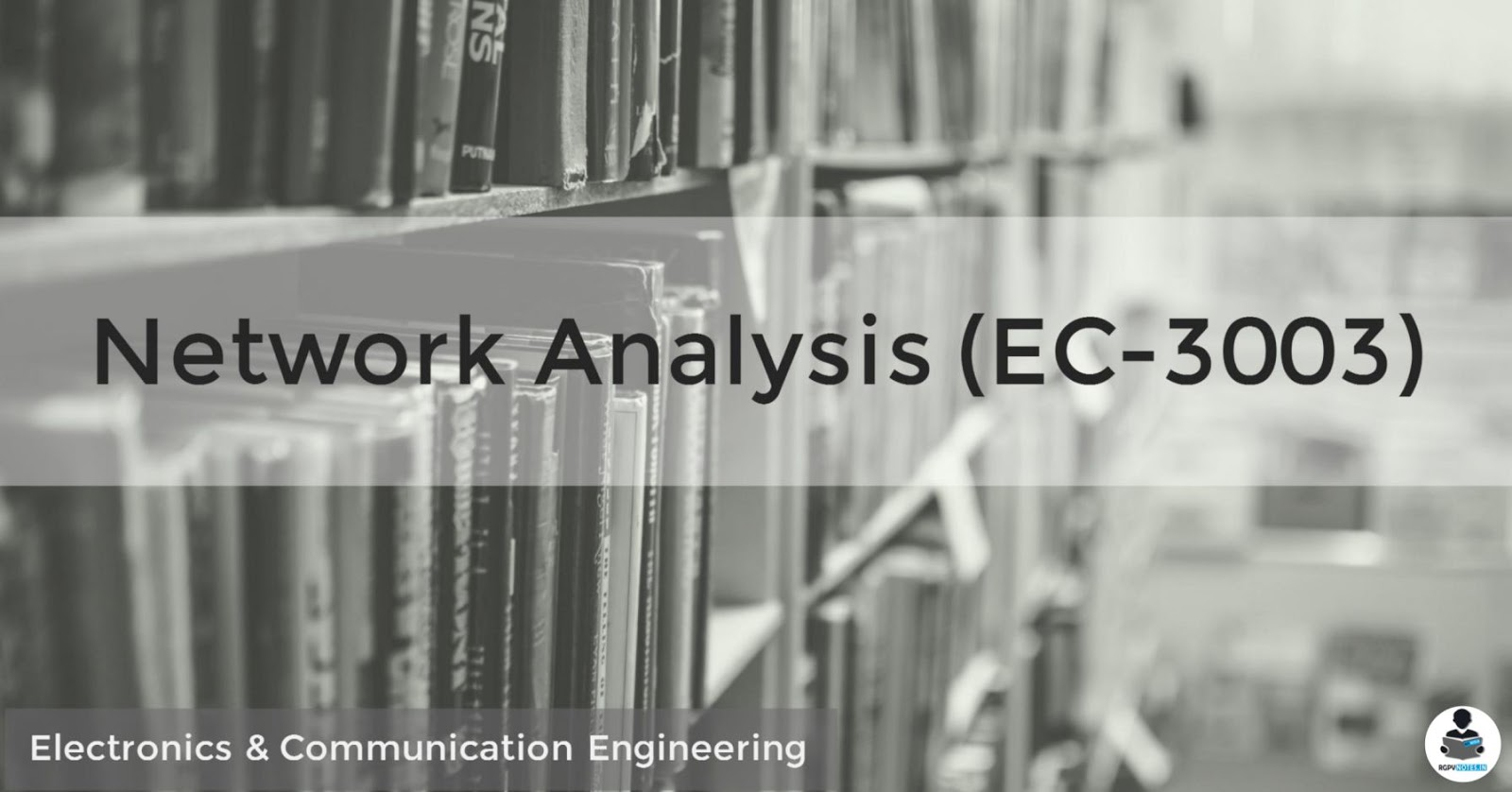### Network Analysis (EC-3003)## Objectives

To make the students capable of analyzing any given electrical network.
To make the students learn how to synthesize an electrical network from a given impedance/admittance function.
Understand the fundamental concepts and theories about networks. - Apply this knowledge to solve real-world, network-centric problems. - Use advanced network analysis methods and tools to visualize and analyze networks. Interpret the results with respect to exploratory, quantitative and substantive questions. - Design and execute a small-scale network analysis project in a systematic fashion.

## Syllabus

UNIT 1:basic circuit element R,L,C and their characteristics in terms of linearity & time dependant nature, voltage & current sources, controlled & uncontrolled sources KCL and KVL analysis, Concept of phasor & vector, impedance & admittance, Nodal & mesh analysis, analysis of magnetically coupled circuits. Dot convention, coupling coefficient, tuned circuits, Series & parallel resonance.

UNIT 2: Concept of Network graph, Tree, Tree branch & link, Incidence matrix, cut set and tie set matrices, dual networks.

UNIT 3: Thevenins & Norton’s, Super positions, Reciprocity, Compensation, Substitution, Maximum power transfer, and Millman’s theorem, Tellegen’s theorem, problems with dependent & independent sources.

UNIT 4: Transients in RL, RC&RLC Circuits, initial& final conditions, time constants. Steady state analysis

UNIT 5: solution of Integro-differential equations, transform of waveform synthesized with step ramp, Gate and sinusoidal functions, Initial & final value theorem, Network Theorems in transform domain.

UNIT 6:Z, Y, ABCD, Hybrid parameters, their inverse & image parameters, relationship between parameters, Interconnection of two ports networks, Reciprocity and Symmetry in all parameter.

• Unit 1
• Unit 2
• Unit 3
• Unit 4
• Unit 5

## Outcomes

Apply the fundamental concepts in solving and analyzing different Electrical networks
Select appropriate and relevant technique for solving the Electrical network in different conditions
Apply mathematics in analyzing and synthesizing the networks in time and frequency domain
Estimate the performance of a particular network from its analysis

## References

1. Sudhakar-Circuit Network Analysis & Synth(TMH).
2. J. David Irwin Engineering Circuit analysis tenth edition, Wiley india.
3. Kuo- Network Analysis & Synthesis, Wiley India.
4. Robert L Boylestad introductory Circuit analysis, Pearson
5. Smarajit Ghosh, NETWORK THEORY: ANALYSIS AND SYNTHESIS (PHI).
6. Roy Choudhary D; Network and systems; New Age Pub.
7. Bhattacharya and Singh- Network Analysis & Synth (Pearson).

## List of Experiments

1. To Verify Thevenin Theorem and Superposition Theorem.
2. To Verify Reciprocity Theorem and Millman’s Theorem.
3. To Verify Maximum Power Transfer Theorem.
4. To Determine Open Circuit and Short Circuit parameters of a Two Port Network.
5. To Determine A,B, C, D parameters of a Two Port Network.
6. To determine h parameters of a Two Port Network.
7. To Find Frequency Response of RLC Series Circuit RLC parallel Circuit and determine resonance and 3dB frequencies.
8. To determine charging and discharging times of Capacitors.